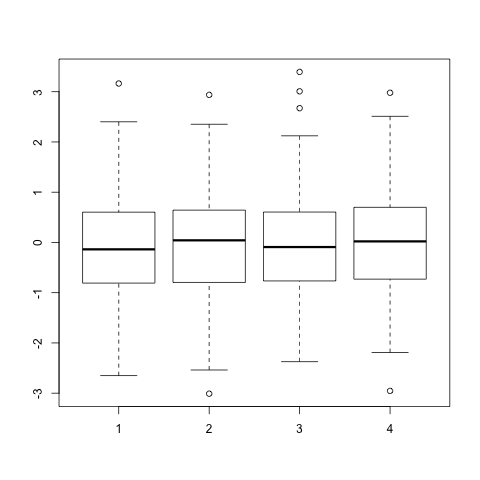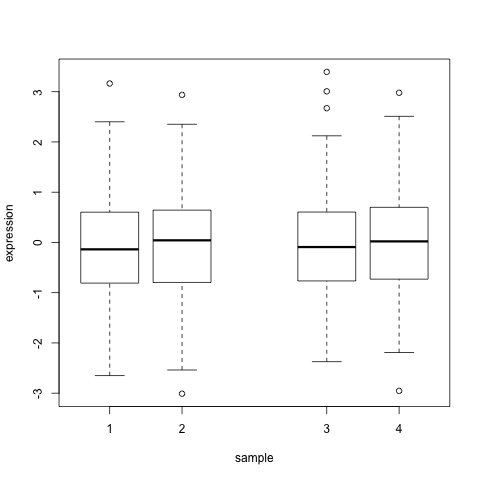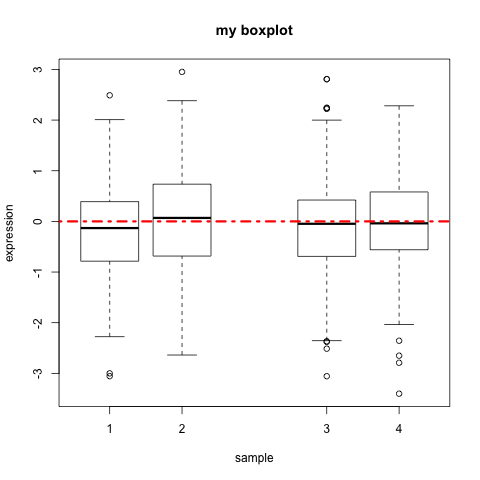## 16.4 Box plots

A boxplot is a convenient way to describe the distribution of the data.

• A simple boxplot:
``````# Create a matrix of 1000 random values from the normal distribution (4 columns, 250 rows)
x <- matrix(rnorm(1000), ncol=4)

# Basic boxplot
boxplot(x)``````• xlab: x-axis label
• ylab: y-axis label
• at: position of each box along the x-axis: here we skip position 3 to allow more space between boxes 1/2 and 3/4
``````boxplot(x,
xlab="sample",
ylab="expression",
at=c(1, 2, 4, 5))``````• Add an horizontal line at y=0 with abline(); arguments of abline :
• h : y-axis starting point of horizontal line (v for a vertical line)
• col : color
• lwd : line thickness
• lty : line type
``````# First plot the box plot as before:
boxplot(x,
xlab="sample",
ylab="expression",
at=c(1, 2, 4, 5),
main="my boxplot")

# Then run the abline function
abline(h=0, col="red", lwd=3, lty="dotdash")``````• Line types in R: